# Pulse Modulation

Pulse modulation is a type of modulation in which the signal is transmitted in the form of pulses. It can be used to transmit analogue information. In pulse modulation, continuous signals are sampled at regular intervals.

Pulse modulation can be classified into two major types.

• Analogue: Indication of sample amplitude is infinitely variable
• Digital: Indicates sample amplitude at the nearest predetermined level.

A block diagram showing the basic classification of modulation techniques is given below.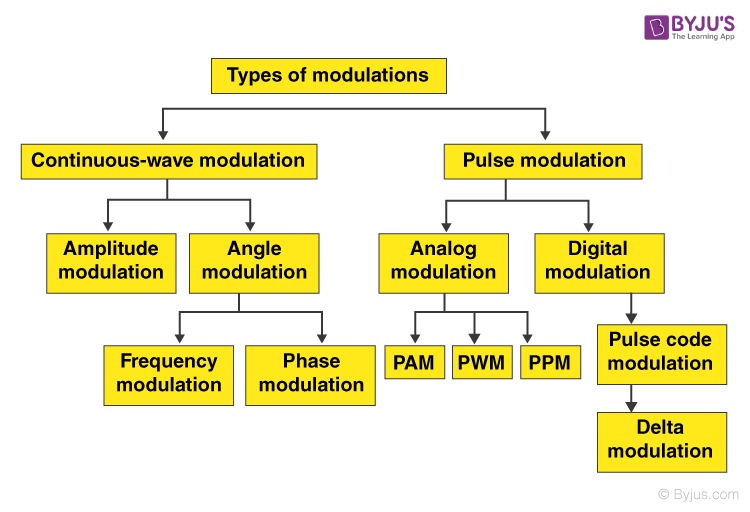## Types of Pulse modulation

Lets us look at some of the different types of pulse modulation.

### Pulse Amplitude Modulation (PAM)

It is the simplest form of Pulse Modulation. In this type of modulation, each sample is made proportional to the amplitude of the signal at the instant of sampling. The PAM signal follows the amplitude of the original signal, as the signal traces out the path of the whole wave. Here a signal which is sampled at Nyquist rate can be reconstructed by passing it through an efficient Low Pass Filter (LPF) with exact cutoff frequency. It is very easy to generate and demodulate PAM. This technique transmits the data by encoding in the amplitude of a series of signal pulses.

There are two types of PAM.

1. Single Polarity PAM: A fixed DC level is added to the signal so that the signal is always positive.
2. Double Polarity PAM: Here the pulses are both positive and negative.

PAM is illustrated in the figure below.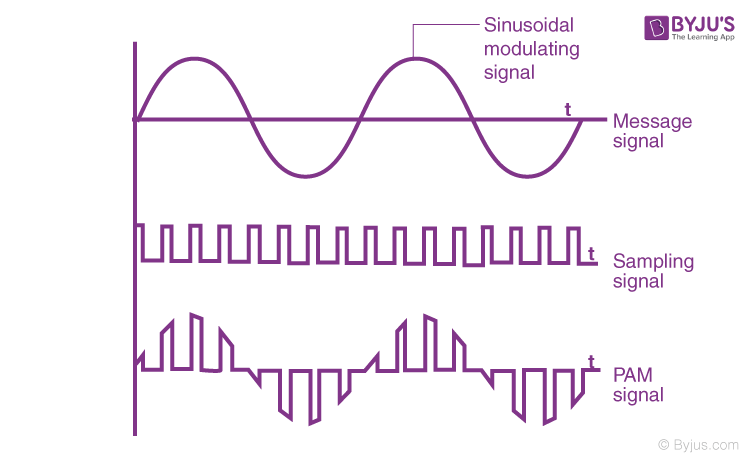From the figure, it is clear that the pulse amplitude modulated signal is following the amplitude of the message signal.

• Both Modulation and demodulation are simple.
• Easy construction of transmitter and receiver circuits.

• Large bandwidth is required for transmission.
• More noise.
• Here the amplitude is varying. Therefore, the power required will be more.

Applications of PAM

• Mainly used in Ethernet communication.
• Many microcontrollers use this technique in order to generate control signals.
• It is used in Photo-biology.
• It acts as an electronic driver for LED circuits.

### Pulse Time Modulation (PTM)

Here the pulses will have the same amplitude. However, one of their timing characteristics is made proportional to the amplitude of the sampled signal. This variable characteristic can be either frequency, position or width. This way pulse time modulation can be classified into three types.

Pulse Width Modulation

Pulse Width Modulation is also known as pulse duration modulation (PDM). Here, as the name suggests, the width of the pulse is varied in proportional to the amplitude of the signal. Since the width is changing, the power loss can be reduced when compared to PAM signals.From the figure, it is clear that the amplitude of the signal is constant. Amplitude limiters are used for this. Since clipping of amplitude at desired levels take place, this modulation produces less noise.

• Low power consumption.
• It has an efficiency of about 90 per cent.
• Noise interference is less.
• High power handling capacity.

• The circuit is more complex.
• Voltage spikes can be seen.
• The system is expensive as it uses semiconductor devices.
• Switching losses will be more due to high PWM frequency.

Applications of PTM

• Used in encoding purposes in the telecommunication system.
• Used to control brightness in a smart lighting system.
• Helps to prevent overheating in LED’s while maintaining it’s brightness.
• Used in audio and video amplifiers.

Pulse Position Modulation

In this type of modulation, both the amplitude and width of the pulse are kept constant. We vary the position of each pulse with reference to a particular pulse. Here a single pulse is transmitted with the required number of phase shifts. So we can say that pulse position modulation is an analogue modulation scheme where the amplitude and width of the pulse are kept constant, while the position of the pulse with respect to the position of a reference pulse is varied according to the instantaneous value of message signal.

PPM can be obtained from PWM. This is done by getting rid of leading-edge and bodies of PWM pulses. The main advantage of pulse position modulation is that it requires constant transmitter power output while the major disadvantage is that it depends upon transmitter-receiver synchronisation.

The waveforms of PPM are given below: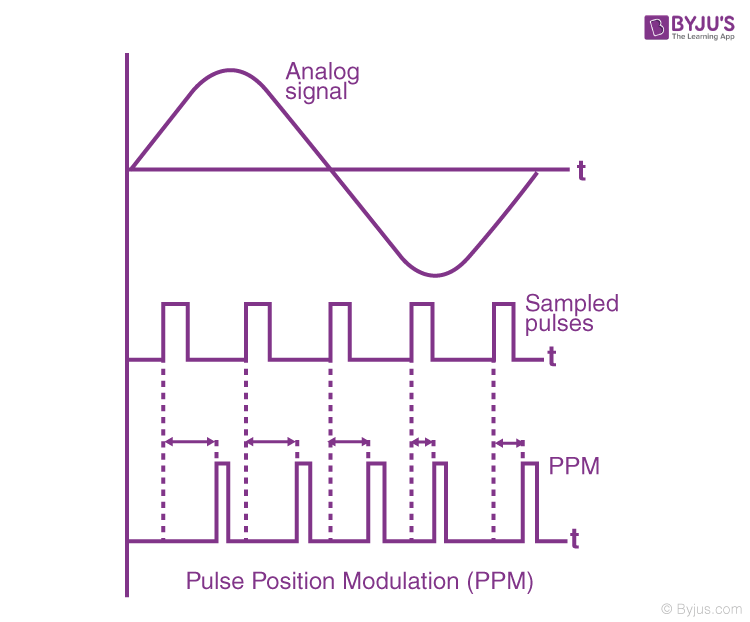However, the third type, pulse-frequency modulation is not used.

• As it has constant amplitude noise interference is less.
• We can easily separate signal from a noisy signal.
• Among all three types, it has the most power efficiency.
• Requires less power when compared to pulse amplitude modulation.

• The system is highly complex.
• The system requires more bandwidth.

Applications of PPM

• It is used in the air traffic control system and telecommunication systems.
• Remote controlled cars, planes, trains use pulse code modulations.
• It is used to compress data and hence it is used for storage.

### Pulse Code Modulation (PCM)

This type of modulation is different from all modulations learnt so far. It is clear from the block diagram given at the top that it is a type of digital modulation. That is the signals here are sampled and sent in pulse form. A common feature among other techniques is that pulse code modulation also uses sampling technique. In this case, instead of sending a pulse train which is capable of continuously varying parameters, this type of generator produces a series of numbers or digits. Each digit in it represents the appropriate length of the sample at a particular instant.

Basic block diagram of its realisation is given below: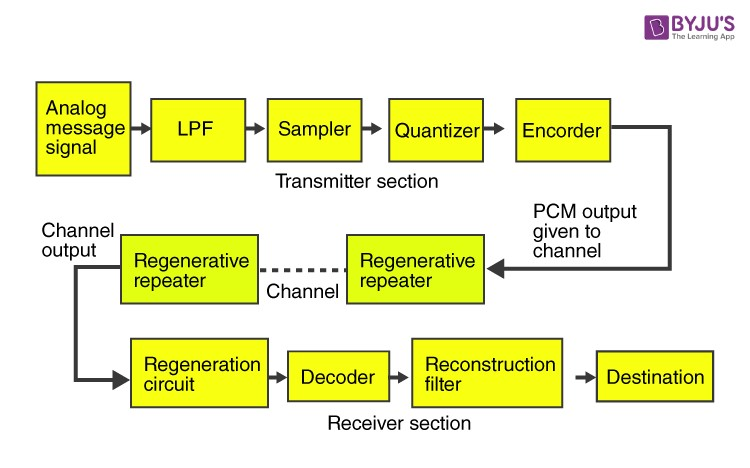• It is mainly used in long distant communication.
• Transmitter efficiency is more.
• It has higher noise immunity when compared to other methods.

• More bandwidth is required when compared to analogue systems.
• In this method encoding, decoding and quantisation of the circuit have to be done. This makes it more complex.

Applications of PCM

• It is used in the satellite transmission system.
• It is also used in space communication.
• Used in Telephony.
• One of the recent applications is the compact disc.

### Delta Modulation

In this type of modulation, the sampling rate is very high. Here, stepsize after quantisation is of the smaller value. In this method, the quantisation design is very simple. Here the bit rate can be designed by the user.

Block diagram of a delta modulator is given below: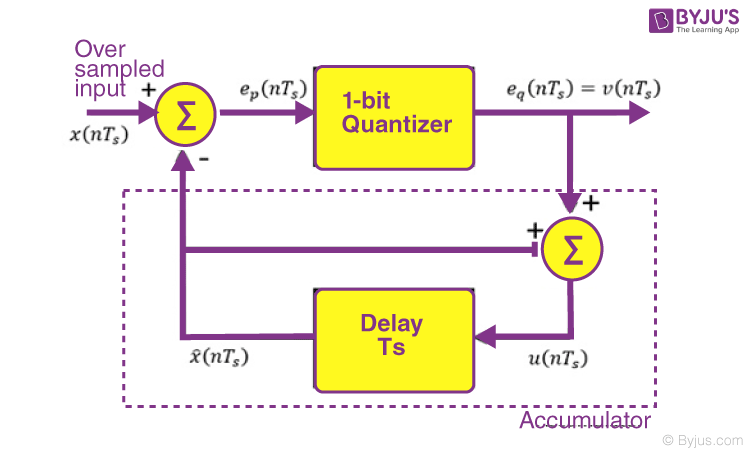Test your Knowledge on Pulse modulation